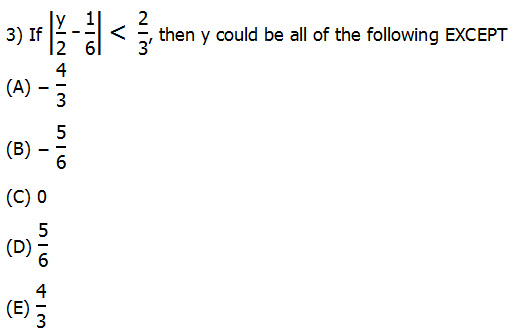GMAT Question of the Day - Daily to your Mailbox; hard ones only

 It is currently 12 Dec 2019, 00:50### GMAT Club Daily Prep

#### Thank you for using the timer - this advanced tool can estimate your performance and suggest more practice questions. We have subscribed you to Daily Prep Questions via email.

Customized
for You

we will pick new questions that match your level based on your Timer History

Track

every week, we’ll send you an estimated GMAT score based on your performance

Practice
Pays

we will pick new questions that match your level based on your Timer History

#### Not interested in getting valuable practice questions and articles delivered to your email? No problem, unsubscribe here.# If |y /2- 1/6| < 2/3, then y could be all of the following except?

Author Message
TAGS:

### Hide Tags

Current StudentD
Joined: 12 Aug 2015
Posts: 2551
Schools: Boston U '20 (M)
GRE 1: Q169 V154If |y /2- 1/6| < 2/3, then y could be all of the following except?  [#permalink]

### Show Tags

1
1200:00

Difficulty:45% (medium)

Question Stats:68% (01:59) correct32% (01:54) wrongbased on 334 sessions

### HideShow timer Statistics

If $$|\frac{y}{2}- \frac{1}{6}| < \frac{2}{3}$$, then y could be all of the following EXCEPT

A. $$-\frac{4}{3}$$

B. $$-\frac{5}{6}$$

C. 0

D. $$\frac{5}{6}$$

E. $$\frac{4}{3}$$

_________________

Originally posted by stonecold on 14 Jun 2017, 19:05.
Last edited by Bunuel on 14 Jun 2017, 22:10, edited 1 time in total.
Renamed the topic and edited the question.
DirectorV
Joined: 04 Dec 2015
Posts: 734
Location: India
Concentration: Technology, Strategy
WE: Information Technology (Consulting)
Re: If |y /2- 1/6| < 2/3, then y could be all of the following except?  [#permalink]

### Show Tags

3
stonecold wrote:$$|\frac{y}{2} - \frac{1}{6}| < \frac{2}{3}$$

Case 1: $$\frac{y}{2} - \frac{1}{6} <\frac{2}{3}$$
$$\frac{y}{2} < \frac{2}{3} + \frac{1}{6}$$
$$\frac{y}{2} < \frac{4+ 1}{6}$$
$$\frac{y}{2} < \frac{5}{6}$$
$$y<\frac{5}{3}$$

Case 2: $$- (\frac{y}{2} - \frac{1}{6}) < \frac{2}{3}$$
$$-\frac{y}{2} + \frac{1}{6} < \frac{2}{3}$$
$$-\frac{y}{2} < \frac{2}{3} - \frac{1}{6}$$
$$-\frac{y}{2} < \frac{4 - 1}{6}$$
$$-\frac{y}{2} < \frac{3}{6}$$
$$-\frac{y}{2} < \frac{1}{2}$$
$$-y < 1$$
$$y > -1$$

Value of $$y ==> -1 < y < \frac{5}{3}$$

Values which come between this range are $$-\frac{5}{6}, 0, \frac{5}{6}, \frac{4}{3}.$$

Therefore y cannot be $$-\frac{4}{3}$$. Answer A...
Math ExpertV
Joined: 02 Sep 2009
Posts: 59685
Re: If |y /2- 1/6| < 2/3, then y could be all of the following except?  [#permalink]

### Show Tags

1
stonecold wrote:
If $$|\frac{y}{2}- \frac{1}{6}| < \frac{2}{3}$$, then y could be all of the following EXCEPT

A. $$-\frac{4}{3}$$

B. $$-\frac{5}{6}$$

C. 0

D. $$\frac{5}{6}$$

E. $$\frac{4}{3}$$

Similar question: https://gmatclub.com/forum/if-y-1-2-11- ... 36878.html
_________________
Target Test Prep RepresentativeG
Affiliations: Target Test Prep
Joined: 04 Mar 2011
Posts: 2809
Re: If |y /2- 1/6| < 2/3, then y could be all of the following except?  [#permalink]

### Show Tags

stonecold wrote:
If $$|\frac{y}{2}- \frac{1}{6}| < \frac{2}{3}$$, then y could be all of the following EXCEPT

A. $$-\frac{4}{3}$$

B. $$-\frac{5}{6}$$

C. 0

D. $$\frac{5}{6}$$

E. $$\frac{4}{3}$$

For this absolute value problem, we have two cases to consider: when (y/2 - ⅙) is positive and when (y/2 - ⅙) is negative. Let’s start with the positive case:

Case 1: (y/2 - ⅙) is positive

y/2 - 1/6 < 2/3

Multiplying by 6, we have:

3y - 1 < 4

3y < 5

y < 5/3

Case 2: (y/2 - ⅙) is negative

-(y/2 - ⅙) < 2/3

-y/2 + 1/6 < ⅔

Multiplying by 6, we have:

-3y + 1 < 4

-3y < 3

y > -1

Thus, -1 < y < 5/3. Thus, y cannot be -4/3.

_________________

# Jeffrey Miller

Jeff@TargetTestPrep.com

See why Target Test Prep is the top rated GMAT quant course on GMAT Club. Read Our Reviews

If you find one of my posts helpful, please take a moment to click on the "Kudos" button.

Non-Human UserJoined: 09 Sep 2013
Posts: 13744
Re: If |y /2- 1/6| < 2/3, then y could be all of the following except?  [#permalink]

### Show Tags

Hello from the GMAT Club BumpBot!

Thanks to another GMAT Club member, I have just discovered this valuable topic, yet it had no discussion for over a year. I am now bumping it up - doing my job. I think you may find it valuable (esp those replies with Kudos).

Want to see all other topics I dig out? Follow me (click follow button on profile). You will receive a summary of all topics I bump in your profile area as well as via email.
_________________Re: If |y /2- 1/6| < 2/3, then y could be all of the following except?   [#permalink] 19 Mar 2019, 09:21
Display posts from previous: Sort by

# If |y /2- 1/6| < 2/3, then y could be all of the following except?